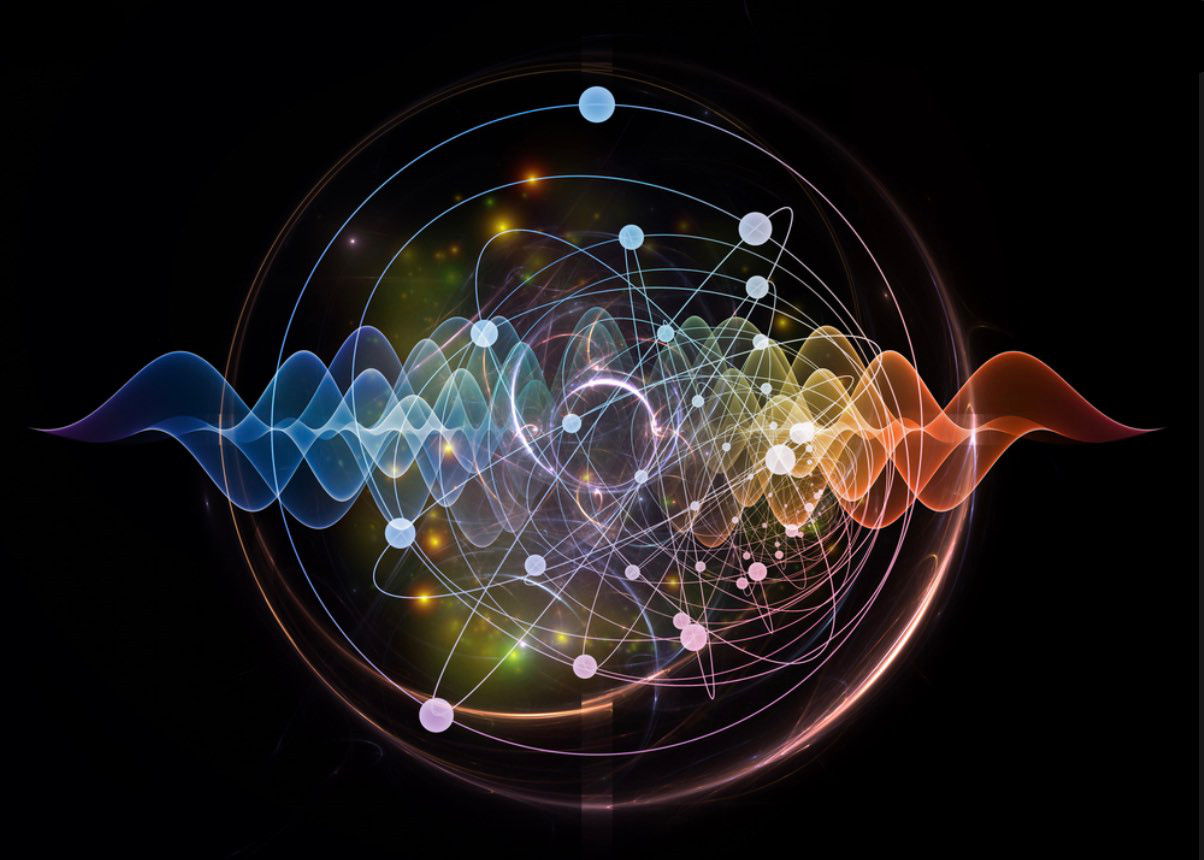## QUANTUM MECHANICS TALK NOTES

INTRODUCTION
A BIT OF HISTORY

Quantum Mechanics is just over 100 years old. Experiments began to punch holes in Physics theory about 1900.
note the big divide
ENERGY COMES IN PACKETS (QUANTA)

THE SIZE OF QUANTA (PARTICLES) - EARLY DISCOVERY This was first discovered by Max Plank to make some experimental data about energy match a ‘new’ theory. He did not like what he found.
The Newtonian theory begins to break down
LIGHT HAS A PARTICLE NATURE

OOPS - EINSTEIN SEES THAT LIGHT HAS A PARTICLE NATURE. Light was thought to be a wave before Einstein's discovery. He made his discovery to make the emerging theory match the experimental data. WON THE NOBEL PRIZE for this.
Light was thought to be a wave
DOUBLE SLIT EXPERIMENT

ANOTHER EXPERIMENT - DEFIES NEWTONIAN PHYSICS: Electrons exhibit both a particle and wave properties ??? What is going on?
Not either ... it's an electron
DEFRACTION

ELECTRONS DEFRACT LIKE WAVES IN A POND!
Oh my!
WAVE INTERFERENCE

HOW WAVES PRODUCE INTERFERENCE PATTERNS: : Like water waves in a pond going through two slits in a barrier.
A WORLD OF PROBABILITES

OMG - ANOTHER EXPERIMENT THAT ASTOUNDS: This one is a killer - we cannot measure things exactly. We are living in a world of probabilites.
We must have a new PhysicsWHAT QUANTUM MECHANICS IS

THE THREE PRINCIPALS: With these three principals all of the wierd experimental data can be explained by a NEW THEORY (Quantum Mechanics)
In a nutshell
WHAT QUANTUM MECHANICS IS

THE SCHRODINGER EQUATION: (WAVE EQUATION): This equation when solved for certain initial conditions yields a wave like those shown below the eqation. This is actually in mathematical terms a pretty simple formula.
The whole ball of wax is contained in this equation
SUPERPOSITION

THE BEATING HEART OF QUANTUM MECHANICS: SUPERPOSITION. This is IT!!!!!! The theory of SUPERPOSITION of waves. That when ever there are two possible states (configurations) the states add together and that also becomes a possible outcome.
The + sign is the usual meaning of addition except that the numbers are complex (another talk sometime).
The beating heart of Quantum Physics
A SAMPLE SOLUTION OF THE SCHRODINGER EQUATION

SOLVING THE SCHRODINGER EQUATION FOR THE NEXT THREE PAGES. Here I solve the Schrodinger equation for a particle moving back and forth in a little box. This is to give you some idea of what Quantum researchers spend their time doing. Cranking away at the Schrodinger equation.
This is to give you some idea of what Quantum researchers spend their time doing. Cranking away at the Schrodinger equation.
CRANKING THE MATH

CRANKING… The math here is not very complicated - except for the fact that the equation is a differential equation, most high school students these days can follow the flow of this computation.
lots of high school algebra just cranking
SOLVED!

STILL CRANKING: AND WE FIND THAT THERE ARE PLACES WHERE THE PARTICLE CAN NEVER BE - TOTALLY CRAZY!!! We also see that the energy levels are quantized and that there can be no zero energy level and that there are places where the particle can never be in the box.
there are places where the particle can never be in the box!!!SUMMARY

QUANTA,
WAVE EQUATION,
SUPERPOSITION,
AND A UNIVERSE THAT IS PROBABLE.
As of now experiments and theory match (the theory even predicts new experiments). In a probable universe there is room for God to intervene, and room for miracles. In my view, science and religion are no longer at odds.
Room for God